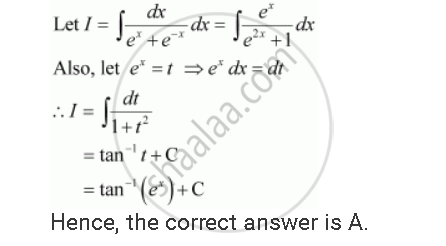Share

# Choose the Correct Int Dx/(E^X + E^(-x)) is Equal to - CBSE (Commerce) Class 12 - Mathematics

ConceptDefinite Integral as the Limit of a Sum

#### Question

Choose the correct int dx/(e^x + e^(-x)) is equal to

(A) tan–1 (ex) + C

(B) tan–1 (e–x) + C

(C) log (ex – e–x) + C

(D) log (ex + e–x) + C

#### SolutionIs there an error in this question or solution?

#### Video TutorialsVIEW ALL 

Solution Choose the Correct Int Dx/(E^X + E^(-x)) is Equal to Concept: Definite Integral as the Limit of a Sum.
S